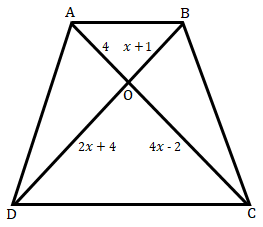"
">

# In figure below, if $AB\ ‚ą•\ CD$, find the value of $x$."

Given;

In the given figure, $AB\ ‚ą•\ CD$.

To do:

We have to find the value of $x$.

Solution:

We know that,

Diagonals of a Trapezium divide each other proportionally.

Therefore,

$\begin{array}{l} \frac{AO}{CO} =\frac{BO}{DO}\\ \\ \frac{4}{4x-2} =\frac{x+1}{2x+4}\\ \\ 4\times ( 2x+4) =( x+1)( 4x-2)\\ \\ 8x+16=x( 4x-2) +1( 4x-2)\\ \\ 8x+16=4x^{2} -2x+4x-2\\ \\ 4x^{2} +2x-8x-2-16=0\\ \\ 4x^{2} -6x-18=0\\ \\ 2\left( 2x^{2} -3x-9\right) =0\\ \\ 2x^{2} -3x-9=0\\ \\ 2x^{2} -6x+3x-9=0\\ \\ 2x( x-3) +3( x-3) =0\\ \\ ( x-3)( 2x+3) =0\\ \\ x-3=0\ \ \ or\ \ 2x+3=0\\ \\ x=3\ \ \ \ or\ \ \ 2x=-3\\ \\ x=3\ \ \ or\ \ \ \ x=\frac{-3}{2} \end{array}$

$x=\frac{-3}{2}$ is not possible because the length cannot be negative.

Therefore,

The value of $x$ is $3$.

Updated on: 10-Oct-2022

28 Views